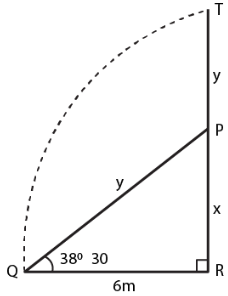Guru

# The upper part of a tree broken by wind falls to the ground without being detached. The top of the broken part touches the ground at an angle of 38° 30’ at a point 6 m from the foot of the tree. Calculate (i) the height at which the tree is broken. (ii) the original height of the tree correct to two decimal places.

• 1

sir this is the  question from the book -ML aggarwal( avichal publication) class 10th , chapter20, heights and distances ……..
we have given that The upper part of a tree broken by wind falls to the ground without being detached. The top of the broken part touches the ground at an angle of 38degree 30’ at a point 6 m from the foot of the tree.
we have to Calculate (i) the height at which the tree is broken. (ii) the original height of the tree correct to two decimal places.
ML Aggarwal ICSE, Heights and distances

Share

1. This answer was edited.

Consider TR as the total height of the tree

TP as the broken part which touches the ground at a distance of 6 m from the foot of the tree which makes an angle of 380 30’ with the ground

Take PR = x and TR = x + y

PQ = PT = yIn right triangle PQR

tan θ = PR/QR

Substituting the values

tan 380 30’ = x/6

x/6 = 0.7954

By cross multiplication

x = 0.7954 × 6 = 4.7724

We know that

sin θ = PR/PQ

Substituting the values

sin 380 30’ = x/y

So we get

0.6225 = 4.7724/y

y = 4.7724/0.6225 = 7.6665

Here

Height of the tree = 4.7724 + 7.6665 = 12.4389 = 12.44m

Height of the tree at which it is broken = 4.77 m

• 4